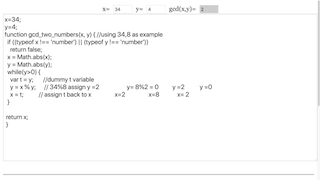### Translations

Code Language Translator Run### Software Requirements

SoftwareRequirements

 Android iOS Windows MacOS with best with Chrome Chrome Chrome Chrome support full-screen? Yes. Chrome/Opera No. Firefox/ Samsung Internet Not yet Yes Yes cannot work on some mobile browser that don't understand JavaScript such as..... cannot work on Internet Explorer 9 and below

### CreditsThis email address is being protected from spambots. You need JavaScript enabled to view it.; Francisco Esquembre; Felix J. Garcia Clemente

### Sample Learning Goals Mathematical solution:

If two numbers, x and y are given, the greatest common divisor of x and y, denoted by gcd(x,y) can be found by reducing the numbers to smaller numbers using the following mathematics results

gcd(x,y) = gcd(y,x%y).
Hence, gcd(34, 8) = gcd(8, 2) = gcd(2,0) = 2

### For Teachers Computing Solution

function gcd_two_numbers(x, y) { //using 34,8 as example
if ((typeof x !== 'number') || (typeof y !== 'number'))
return false;
x = Math.abs(x);
y = Math.abs(y);
while(y>0) {  // do this while y is greater than 0, in other words remainder of x%y is non-zero
var t = y; //dummy t variable
y = x % y; // 34%8 assign y =2 y= 8%2 = 0 y =2 y =0

x = t; // assign t back to x x=2 x=8 x= 2
}
return x;  //  return as output when the algorithm stops

}

Research

[text]

[text]

[text]